## Brocard Triangles

Let the point of intersection ofandbe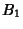, where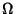andare the Brocard Points, and similarly defineand.is the first Brocard triangle, and is inversely similar to. It is inscribed in the Brocard Circle drawn withas the Diameter. The triangles,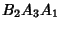, andare Isosceles Triangles with base angles, whereis the Brocard Angle. The sum of the areas of the Isosceles Triangles is, the Area of Triangle. The first Brocard triangle is in perspective with the given Triangle, with,, andConcurrent. The Median Point of the first Brocard triangle is the Median Pointof the original triangle. The Brocard triangles are in perspective at.

Let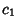,, and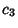and,, andbe the Circles intersecting in the Brocard Pointsand, respectively. Let the two circlesandtangent attoand, and passing respectively through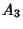and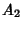, meet again at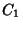. The triangleis the second Brocard triangle. Each Vertex of the second Brocard triangle lies on the second Brocard Circle.

The two Brocard triangles are in perspective at.